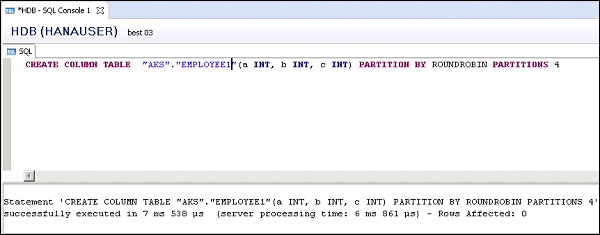# SAP HANA Admin - Table Partition

Using Partitioning, you can split column store tables into smaller tables in a multiple host system. You can even divide tables into smaller more manageable parts using partitioning. Partitioning can be done using DML SQL statements.

When a table is partitioned it contains different set of rows for each part and Partitioning can be done based on different algorithms - Hash Partitioning, Single Level or multilevel Partitioning.

Following are the advantages of using Table Partitioning −

### Partitioning Pruning

You can determine if a query can be analyzed in a particular partition. Using this method, you can reduce the load on the system and hence improve the response time.

Example − When you partition a table based on the year, a query is executed to analyze the data for a particular year. You can use specific partition and hence query performance is improved.

You can divide individual partitions on multiple hosts and hence a query is not processed by a single server, results in better load balancing on all the servers hosting table partitions.

### Size Limitation

In a column store table, maximum number of rows that can be entered is around 2 billion. You can overcome this by distributing the rows of a column table on multiple partitions and hence the size limit is increased to 2 billion for each partition.

### Improved Delta Merge Operation

During delta merge, if data is only modified for some partitions, you need to merge less partitions in delta merge.

### Parallel Processing of Queries

Using partitions, you can run multiple queries in parallel and hence improve the response time.

Let us now discuss the types of partionining.

## Single Level Partitioning

There are different types of single level partitioning available in SAP HANA system −

• Hash Partitioning
• Round Robin Partitioning
• Range Partitioning

In Hash partitioning, rows are distributed equally to perform load balancing. You don’t require the detailed information about table content in this Partitioning type.

As compared to Hash Partitioning, in Round Robin Partitioning rows are equally distributed to each partition and new rows are equally assigned to each partition.

To create 4 partitions of a table using Round Robin, you can use the following SQL queries. No primary keys should be defined.

```CREATE COLUMN TABLE TABLENAME (a INT, b INT, c INT)
PARTITION BY ROUNDROBIN PARTITIONS 4
```

This command will create 4 partitions of a table.```CREATE COLUMN TABLE Table_Name (a INT, b INT, c INT, PRIMARY KEY (a,b))
PARTITION BY HASH (a, b) PARTITIONS 4
```

This will create 4 partitions on column a and b, and you need to specify at least one column.

## Range Partitioning

In Range partitioning, you create dedicated partitions for certain values and you should have in-depth knowledge of table contents for partitioning columns.

Example − Creating one partition for each calendar month.

```CREATE COLUMN TABLE TABLE_NAME (a INT, b INT, c INT, PRIMARY KEY (a,b))
PARTITION BY RANGE (a)
(PARTITION 1 <= VALUES < 10, PARTITION 10 <= VALUES < 20,
PARTITION VALUE = 50, PARTITION  OTHERS)
```

This will create 4 partitions, one for partition range 1 to 10, 2nd from 10 to 20, 3rd for value 50, and 4th for all other values.

## Multilevel Partitioning

In multilevel partitioning, you can overcome the limitation of HASH and RANGE single level partitioning to use key columns as partitioning columns. Using multilevel partitioning, you can partition a column that is not a primary key. The most common type of multilevel partitioning is HASH-Range Partitioning.

In Hash-Range multilevel partitioning, you implement Hash partitioning at the first level to implement load balancing and Range partitioning at the second level to implement timebased partitioning.

```CREATE COLUMN TABLE Table_name (a INT, b INT, c INT, PRIMARY KEY (a,b))
PARTITION BY HASH (a, b) PARTITIONS 4,
RANGE (c) (PARTITION 1 <= VALUES < 10, PARTITION 10 <= VALUES < 20)
```

Instead of using Hash partitioning at the first level, you can also use Round Robin partitioning and it is known as Round Robin-Range multilevel partitioning.

```CREATE COLUMN TABLE Table_name (a INT, b INT, c INT)
PARTITION BY ROUNDROBIN PARTITIONS 4,
RANGE (c) (PARTITION 1 <= VALUES < 10, PARTITION 10 <= VALUES < 20)
```